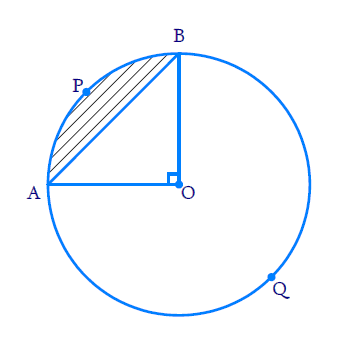# Ex.12.2 Q4 Areas Related to Circles Solution - NCERT Maths Class 10

Go back to  'Ex.12.2'

## Question

A chord of a circle of radius $$10\, \rm{cm}$$ subtends a right angle at the centre. Find the area of the corresponding

(i) minor segment

(ii) major sector. (Use $$\pi = 3.14$$)

Video Solution
Areas Related To Circles
Ex 12.2 | Question 4

## Text Solution

What is known?

Radius of the circle and angle subtended by the chord at the center.

What is unknown?

(i) Area of minor segment

(ii) Area of major segment

Reasoning:

In a circle with radius r and angle at the centre with degree measure $$\theta$$;

(i) Area of the sector\begin{align}= \frac{{\rm{\theta }}}{{360}^{\rm{o}}} \times \pi {r^2}\end{align}

(ii) Area of the segment = Area of the sector- Area of the corresponding triangle.

Area of the triangle\begin{align}= \frac{1}{2} \times \rm base \times height\end{align}

Draw a figure to visualize the area to be found out.Here,\begin{align}{\rm{Radius, }}r = 10\rm \,cm{\rm{\theta }} = {90^{\rm{o}}}\end{align}

Visually it's clear from the figure that;

Let $${AB}$$ is the chord subtends a right angle at the centre.

(i) Area of minor segment $${APB} =$$ $$\text{Area of sector}$$ $${OAPB}\, -\,$$$$\text{Area of right}$$$$\Delta {AOB}$$

(ii) Area of major segment \begin{align}AQB{\rm{ }} = \pi {r^2} - \text{Area of minor segment APB}\end{align}

Area of the right triangle

\begin{align}\Delta AOB = \frac{1}{2} \times OA \times OB\end{align}

\begin{align}&= {\frac{\theta }{{{{360}^\circ }}} \times \pi {r^2}}\\&= {\frac{{{{90}^\circ }}}{{{{360}^\circ }}} \times \pi {r^2}}\\&= {\frac{{\pi {r^2}}}{4}}\end{align}

\begin{align} &= \frac{1}{2} \times {\text{ base }} \times \,\text{height}\\ &= \frac{1}{2} \times {OA} \times {OB}\,\,\,\,\,\,\,\,\,\,\left( {\because {{OA}} \bot {{OB}}} \right)\\& = \frac{1}{2} \times {\text{radius}} \times \rm{radius}\end{align}

Steps:(i) Area of the minor segment $${APB}$$$$=$$Area of sector \begin{align}\rm{OAPB} - \text{ Area of right }{\Delta AOB}\end{align}

\begin{align} & =\left[ \begin{array} & \frac{{{90}^{{}^\circ }}}{{{360}^{{}^\circ }}}\times \pi {{r}^{2}}- \\ \frac{1}{2}\times OA\times OB \\ \end{array} \right]\ \\ & (\because OA\bot OB) \\ & =\frac{\pi {{\text{r}}^{\text{2}}}}{4}-\frac{1}{2}\times r\times r \\ & ={{\text{r}}^{\text{2}}}\left( \frac{\pi }{\text{4}}\text{-}\frac{\text{1}}{\text{2}} \right) \\ & ={{r}^{2}}\left( \frac{3.14-2}{4} \right) \\ & =\left( \frac{{{r}^{2}}\times 1.14}{4} \right) \\ & =\frac{10\times 10\times 1.14}{4}\text{ c}{{\text{m}}^{2}} \\ & =28.5\text{c}{{\text{m}}^{2}} \\ \end{align}

ii) Area of major segment $${AQB} =\pi \rm{r^2}-$$ Area of minor segment $${APB}$$

\begin{align} &= \pi \times 10 \times 10 - 28.5\\&= 3.14 \times 100 - 28.5\\&= 314 - 28.5\\&= 285.5{\text{ cm}^2}\end{align}

Learn from the best math teachers and top your exams

• Live one on one classroom and doubt clearing
• Practice worksheets in and after class for conceptual clarity
• Personalized curriculum to keep up with school